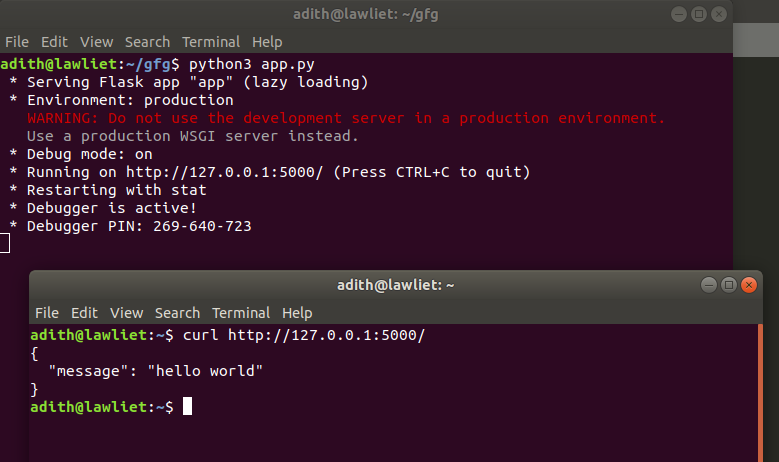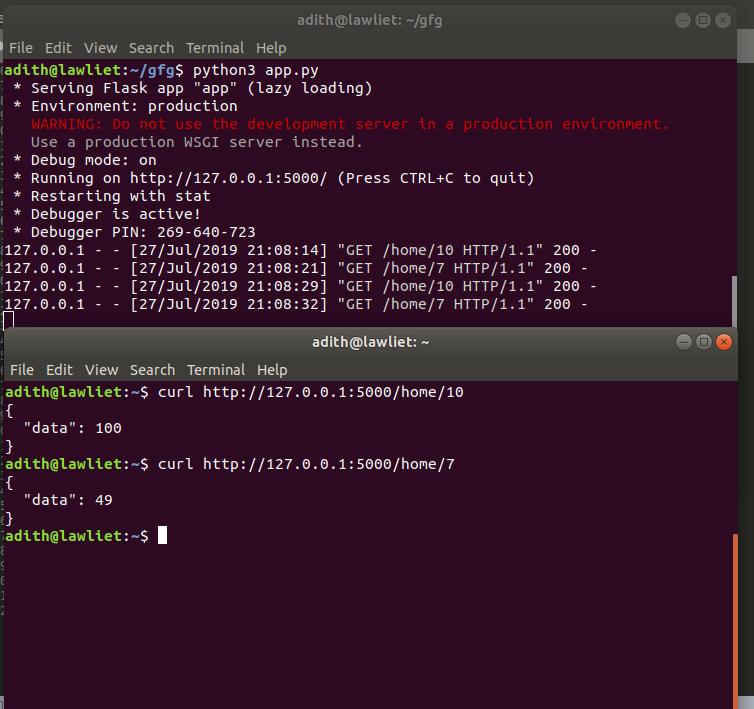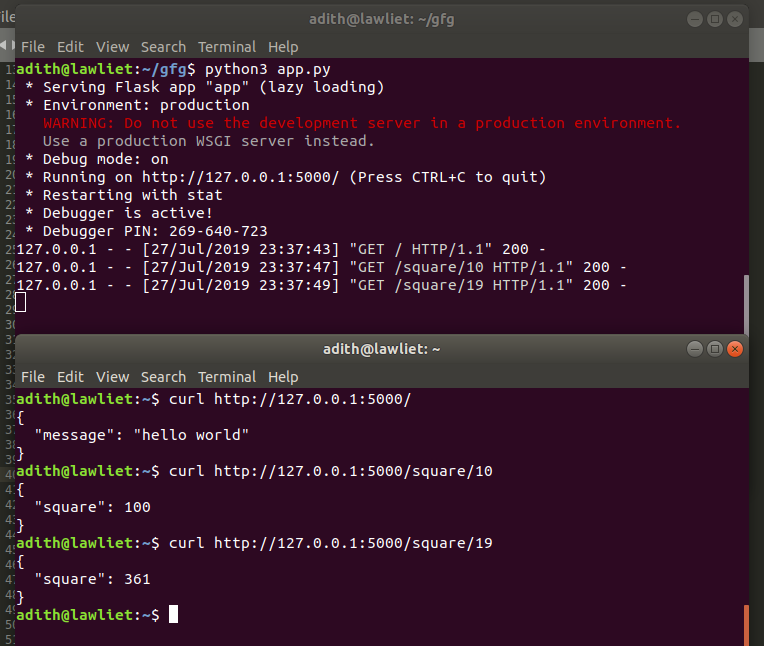# Python | Build a REST API using Flask

• Difficulty Level : Basic
• Last Updated : 25 Feb, 2022

Prerequisite: Introduction to Rest API

REST stands for REpresentational State Transfer and is an architectural style used in modern web development. It defines a set or rules/constraints for a web application to send and receive data.

In this article, we will build a REST API in Python using the Flask framework. Flask is a popular micro framework for building web applications. Since it is a micro-framework, it is very easy to use and lacks most of the advanced functionality which is found in a full-fledged framework. Therefore, building a REST API in Flask is very simple.

There are two ways of creating a REST API in Flask:

1. Using Flask without any external libraries

## Libraries required:

`flask_restful `can be installed via the pip command:

`` sudo pip3 install flask-restful ``

## Method 1: using only Flask

Here, there are two functions: One function to just return or print the data sent through GET or POST and another function to calculate the square of a number sent through GET request and print it.

 `# Using flask to make an api``# import necessary libraries and functions``from` `flask ``import` `Flask, jsonify, request`` ` `# creating a Flask app``app ``=` `Flask(__name__)`` ` `# on the terminal type: curl http://127.0.0.1:5000/``# returns hello world when we use GET.``# returns the data that we send when we use POST.``@app``.route(``'/'``, methods ``=` `[``'GET'``, ``'POST'``])``def` `home():``    ``if``(request.method ``=``=` `'GET'``):`` ` `        ``data ``=` `"hello world"``        ``return` `jsonify({``'data'``: data})`` ` ` ` `# A simple function to calculate the square of a number``# the number to be squared is sent in the URL when we use GET``# on the terminal type: curl http://127.0.0.1:5000 / home / 10``# this returns 100 (square of 10)``@app``.route(``'/home/'``, methods ``=` `[``'GET'``])``def` `disp(num):`` ` `    ``return` `jsonify({``'data'``: num``*``*``2``})`` ` ` ` `# driver function``if` `__name__ ``=``=` `'__main__'``:`` ` `    ``app.run(debug ``=` `True``)`

Output:Executing the square function:Flask Restful is an extension for Flask that adds support for building REST APIs in Python using Flask as the back-end. It encourages best practices and is very easy to set up. Flask restful is very easy to pick up if you’re already familiar with flask.

In `flask_restful`, the main building block is a resource. Each resource can have several methods associated with it such as GET, POST, PUT, DELETE, etc. for example, there could be a resource that calculates the square of a number whenever a get request is sent to it. Each resource is a class that inherits from the Resource class of flask_restful. Once the resource is created and defined, we can add our custom resource to the api and specify a URL path for that corresponding resource.

 `# using flask_restful``from` `flask ``import` `Flask, jsonify, request``from` `flask_restful ``import` `Resource, Api`` ` `# creating the flask app``app ``=` `Flask(__name__)``# creating an API object``api ``=` `Api(app)`` ` `# making a class for a particular resource``# the get, post methods correspond to get and post requests``# they are automatically mapped by flask_restful.``# other methods include put, delete, etc.``class` `Hello(Resource):`` ` `    ``# corresponds to the GET request.``    ``# this function is called whenever there``    ``# is a GET request for this resource``    ``def` `get(``self``):`` ` `        ``return` `jsonify({``'message'``: ``'hello world'``})`` ` `    ``# Corresponds to POST request``    ``def` `post(``self``):``         ` `        ``data ``=` `request.get_json()     ``# status code``        ``return` `jsonify({``'data'``: data}), ``201`` ` ` ` `# another resource to calculate the square of a number``class` `Square(Resource):`` ` `    ``def` `get(``self``, num):`` ` `        ``return` `jsonify({``'square'``: num``*``*``2``})`` ` ` ` `# adding the defined resources along with their corresponding urls``api.add_resource(Hello, ``'/'``)``api.add_resource(Square, ``'/square/'``)`` ` ` ` `# driver function``if` `__name__ ``=``=` `'__main__'``:`` ` `    ``app.run(debug ``=` `True``)`

Output:My Personal Notes arrow_drop_up